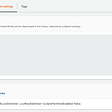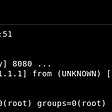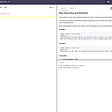# Implementing intefaces from Java world

`public interface Calculator<T> {    int add(T a, T b);}`

# Approach #1: Classic

`// Kotlin Implementation #1class IntegerCalculator : Calculator<Int> {    override fun add(a: Int, b: Int): Int {        return a + b    }}// Invocationval integerCalculator = IntegerCalculator()integerCalculator.add(2, 3)`

# Approach #2: Object expressions

`val integerCalculator = object : Calculator<Int> {    override fun add(a: Int, b: Int): Int {        return a + b    }}print(integerCalculator.add(1, 2))`

# Approach #3: Uncle Sam in Java Interfaces

`val integerCalculator = Calculator<Int> { a, b -> a + b }integerCalculator.add(2, 3)`
`public interface Calculator<T> {    T square(int x);}`

## Object expressions approach

`val integerCalculator = object : Calculator<Int>{    override fun square(x: Int): Int {        return x*x    }}integerCalculator.square(2)`

## The Kotlin Way: Passing in a lambda expression

`val integerCalculator = Calculator { it * it }integerCalculator.square(2)`

# But that doesn’t make sense!

`class Calculator<T>(private val squareOperation: (x: T) -> T) {    fun square(x: T): T {        return squareOperation(x)    }}// Invocationval integerCalculator = Calculator<Int> { x -> x * x }integerCalculator.square(2)`

--

--

--

## More from Amol Pednekar

Building the future, one BLOCK at a time. Blockchain R&D engineer @PersistentSys.

Love podcasts or audiobooks? Learn on the go with our new app.

## Missing Column after Migration to S3 Using DMS## Growth Hacking: How to get (scrape) a product catalogue from an e-commerce site## x64 SLAE — Assignment 2: Reverse Shell## What are Coroutines in Unity?## What is Amazon Elastic Load Balancer (ELB)## Short review of CI/CD tools in 2020## Amol Pednekar

Building the future, one BLOCK at a time. Blockchain R&D engineer @PersistentSys.

## How to launch an Instant app to production on the Google Play Store## Paytm Payment Gateway Integration on Android Studio## Product release: react-channel and adding Kotlin